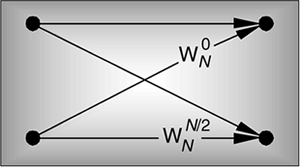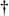# Chapter Four. The Fast Fourier Transform

## Four The Fast Fourier TransformAlthough the DFT is the most straightforward mathematical procedure for determining the frequency content of a time-domain sequence, it's terribly inefficient. As the number of points in the DFT is increased to hundreds, or thousands, the amount of necessary number crunching becomes excessive. In 1965 a paper was published by Cooley and Tukey describing a very efficient algorithm to implement the DFT. That algorithm is now known as the fast Fourier transform (FFT).[] Before the advent of the FFT, thousand-point DFTs took so long to perform that their use was restricted to the larger research and university computer centers. Thanks to Cooley, Tukey, and the semiconductor industry, 1024-point DFTs can now be performed in a few seconds on home computers.

[] Actually, the FFT has an interesting history. While analyzing X-ray scattering data, a couple of physicists in the 1940s were taking advantage of the symmetries of sines and cosines using a mathematical method based on a technique published in the early 1900s. Remarkably, over 20 years passed before the FFT was (re)discovered. Reference  tells the full story.

Volumes have been written about the FFT, and, like no other innovation, the development of this algorithm transformed the discipline of digital signal processing by making the power of Fourier analysis affordable. In this chapter, we'll show why the most popular FFT algorithm (called the radix-2 FFT) is superior to the classical DFT algorithm, present a series of recommendations to enhance our use of the FFT in practice, and provide a list of sources for FFT routines in various software languages. We conclude this chapter, for those readers wanting to know the internal details, with a derivation of the radix-2 FFT and introduce several different ways in which this FFT is implemented.AmazonPrev don't be afraid of buying books Next